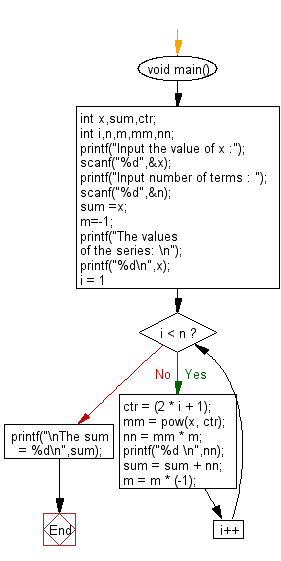﻿ C : Calculate the sum of the series [ x - x^3 + x^5 + …]# C Exercises: Calculate the sum of the series [ x - x^3 + x^5 + ......]

## C For Loop: Exercise-24 with Solution

Write a program in C to find the sum of the series [ x - x^3 + x^5 + ......].

Sample Solution:

C Code:

``````#include <stdio.h>
#include <math.h>
void main()
{
int x,sum,ctr;
int i,n,m,mm,nn;
printf("Input the value of x :");
scanf("%d",&x);
printf("Input number of terms : ");
scanf("%d",&n);
sum =x; m=-1;
printf("The values of the series: \n");
printf("%d\n",x);
for (i = 1; i < n; i++)
{
ctr = (2 * i + 1);
mm = pow(x, ctr);
nn = mm * m;
printf("%d \n",nn);
sum = sum + nn;
m = m * (-1);
}
printf("\nThe sum = %d\n",sum);
}
```
```

Sample Output:

```Input the value of x :2
Input number of terms : 5
The values of the series:
2
-8
32
-128
512

The sum = 410
```

Flowchart:C Programming Code Editor:

Improve this sample solution and post your code through Disqus.

What is the difficulty level of this exercise?

Test your Programming skills with w3resource's quiz.

﻿